# 炒股，我只做强势股 强势股形态大综合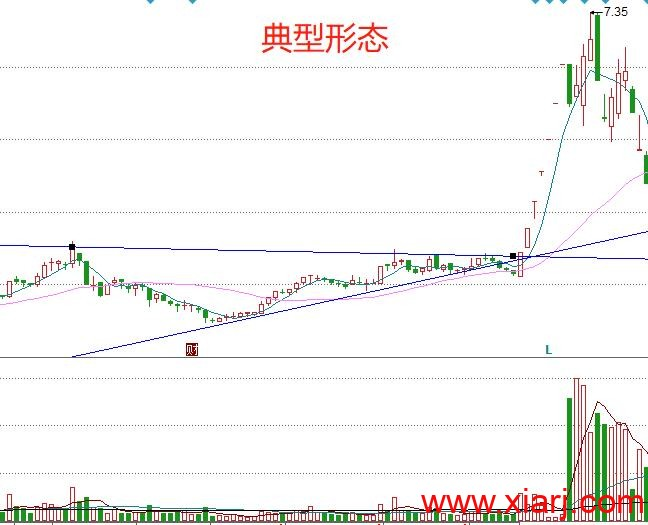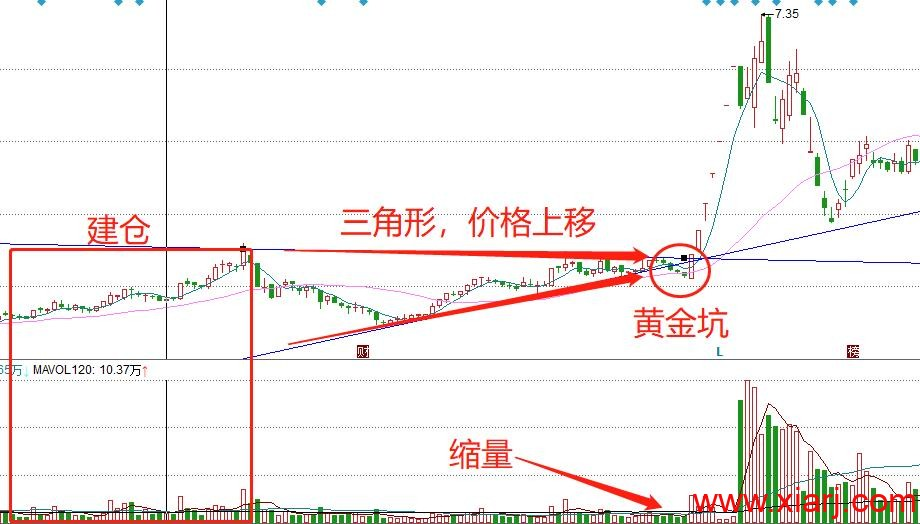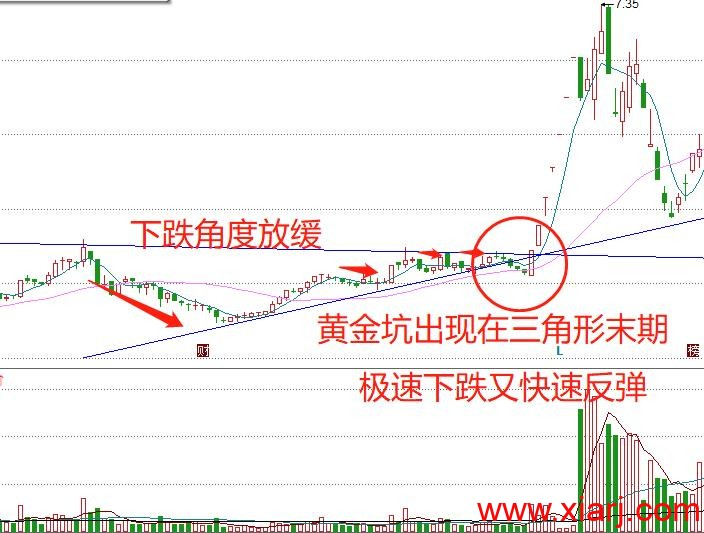1、在上涨趋势中，个股在拉升途中，出现至少一次的天量柱，量能创下一年以来的峰值。这是主力深度介入的信号，普通散户没有办法形成如此巨量。

2、阶段拉升后，出现再次回踩的现象，而且回踩过程中，5日均线拐头向下，10日均线继续保持上扬态势，两根均线交叉，形成老鸭头形态。

3、调整中股价下破了5日均线，但回踩不跌破10日均线，始终保持在10日均线上方，意味着个股能够稳定保持在强势状态，后续有望再迎来新一波行情。

4、入场时机：“天量老鸭头”形态确认后，并且继续保持强势上扬的形态，在股价再次站上5日均线时，及时出手，坐等主力抬轿。

5、止损离场：如果股价无法站稳5日均线上方，重新走弱，说明主力做多意愿不够坚决，后续行情容易反复，止损离场更妥当。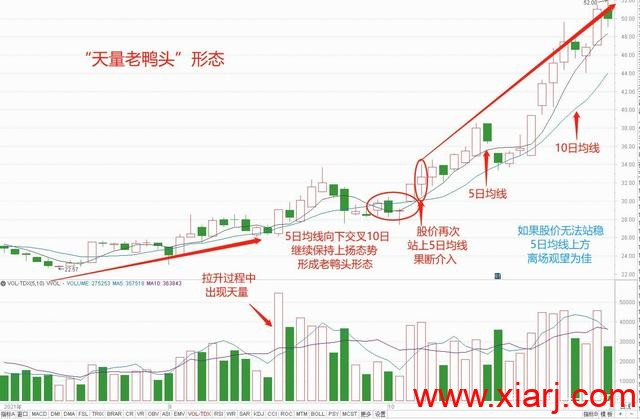1、突破性涨停板

2、反弹性涨停板

3、攻击性涨停板

4、试盘测压性涨停板

5、诱多性涨停板。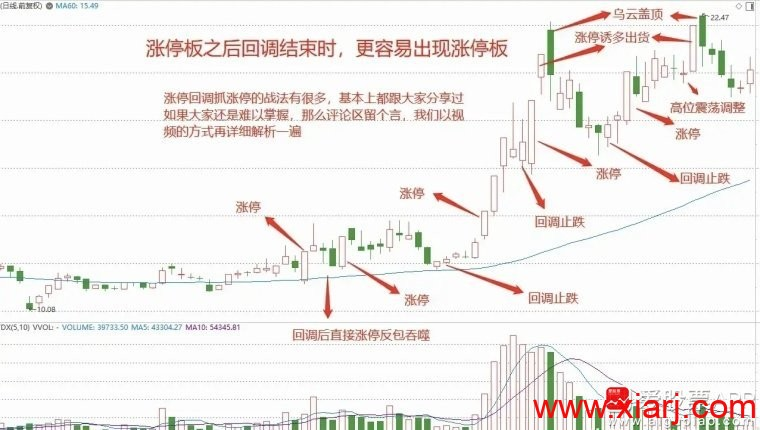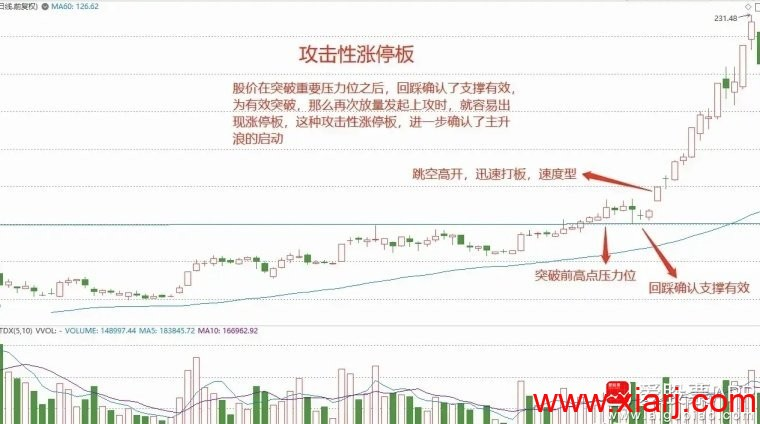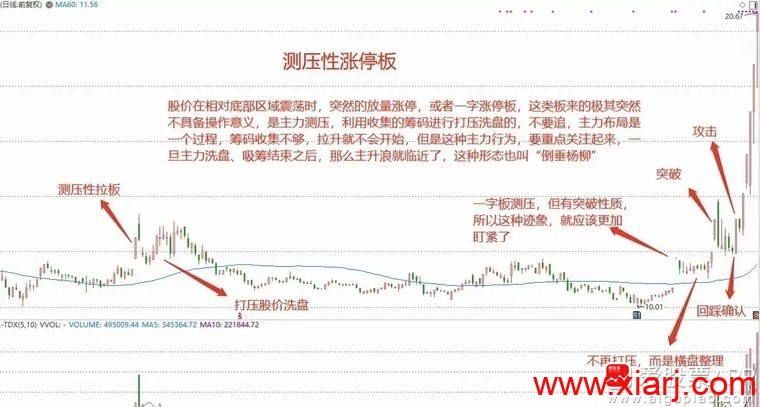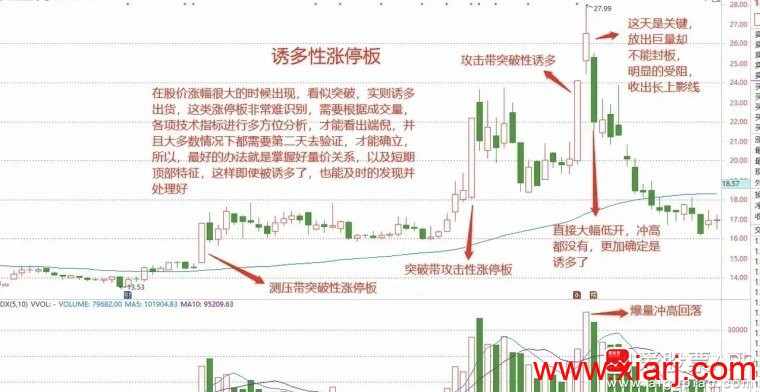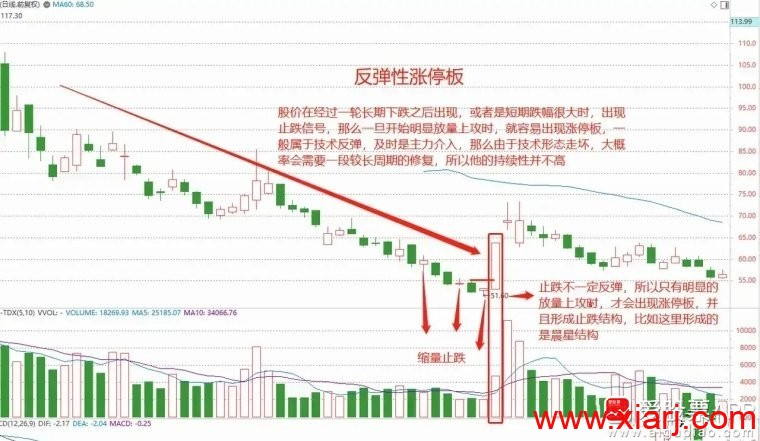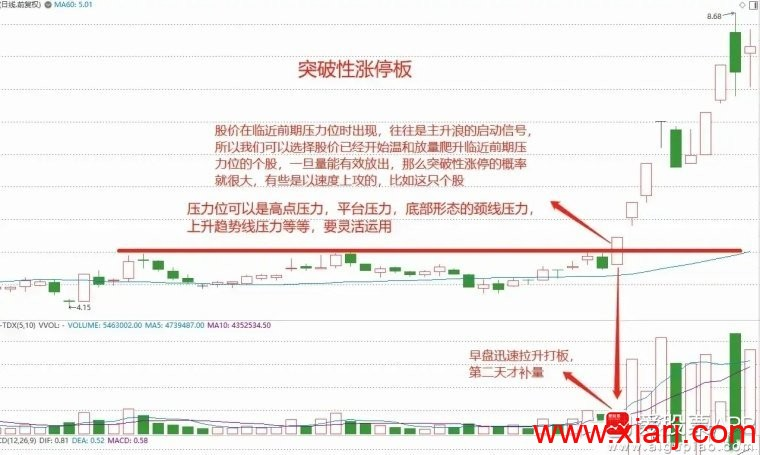Var1:=EMA(SLOPE(C,3)/REF(C,14),14);
Var2:=EMA(SLOPE(INDEXC,3)/REF(INDEXC,14),14);
10000*(Var1-Var2)>88;

AA:=MA(C,N);
BB:=atan((aa-ref(aa,1)))*3.1416*10;

N=10或其它自己设定

x1:=(C L H)/3;
bb13:=atan(ema(x1,13)-ref(ema(x1,13),1))*3.1416*10;
filter(bb13>1 and ema(x1,13)>ref(ema(x1,8),1),10);

A:=(C-REF(O,N))/REF(O,N);
B:=(INDEXC-REF(INDEXO,N))/REF(INDEXO,N);
A>B AND A/B>=2;
N=10或其它自己设定

ys:=(c-l)/(h-l)*100;
x:=hhv(h,5)-ref(o,5);
y:=c-llv(l,5);
z:=(hhv(h,5)-llv(l,5))*2;
bd:=(x y)/z*100;
cross(ys,bd) and bd>ref(bd,1);

aa:=count(c>强命线,3)=3;
bb:=count(c>强阻力,3)=3;
cc:=count(主筹估算>60,3)=3;
dd:=aa and bb and cc;
Var2:=(CLOSE-REF(CLOSE, 1))/REF(CLOSE, 1)*100;
Var3:=(INDEXC-REF(INDEXC, 1))/REF(INDEXC, 1)*100;
Var4:=Var2-Var3;
Var5:=(OPEN-REF(CLOSE, 1))/REF(CLOSE, 1)*100;
Var6:=(INDEXO-REF(INDEXC, 1))/REF(INDEXC, 1)*100;
Var7:=Var5-Var6;
j5:=ema(SUM(REF(Var4, 1), 0) 100 Var7,5);
j10:=ema(SUM(REF(Var4, 1), 0) 100 Var7,10);
j20:=ema(SUM(REF(Var4, 1), 0) 100 Var7,20);
j30:=ema(SUM(REF(Var4, 1), 0) 100 Var7,30);
ee:=j5>j10 and j10>j20 and j20>j30;
dd and ee;

### 最后编辑于：2022/3/22作者：闽发论坛